## Overview

This guide follows on from our blog post that introduces the Arm Compute Library.

The instructions given here show you how to develop a Convolutional Neural Network (CNN) called AlexNet using just the Arm Compute Library and a Raspberry Pi. Links are provided to all of the software tools that you need to get up and running.

The guide starts by introducing Arm Compute Library's graph API and AlexNet, two tools that help simplify developing neural networks on a Raspberry Pi. An example of AlexNet using the graph API, in C++, is explained in detail to help you get started running your own and other classifiers.

By following the steps in this guide, you will be up and running with AlexNet. AlexNet is one of the first Deep Convolutional Neural Networks (CNN) designed to recognize 1000 different object categories within images. You use AlexNet to classify an image of a go-kart with the neural network returning some predictions based on the image content. The network can only be used to predict the 1000 object categories that it has been trained with. If you want to use the CNN for a different task, then the network has to be retrained.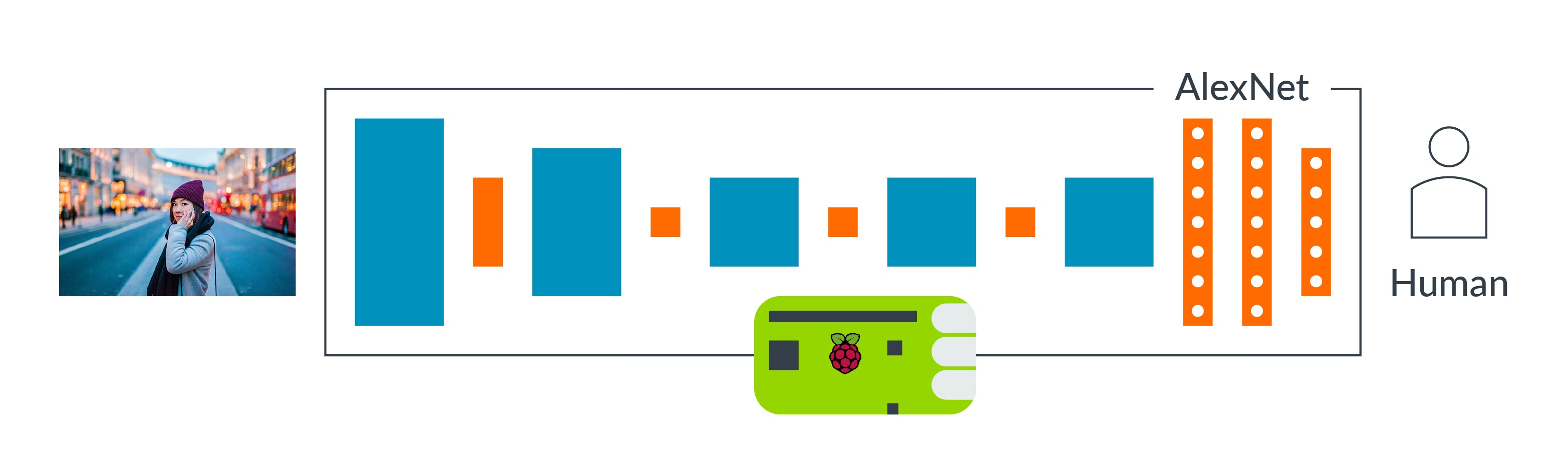## Before you begin

This guide is a sequel to our blog post on how to apply a cartoon effect with the Compute Library. The blog post introduces the Arm Compute Library and provides a simple example of how to use Raspberry with SSH. The post explains how to compile or cross-compile the Arm Compute Library for Raspberry Pi, but this is also covered here.

In addition to some basic knowledge of the Arm Compute Library, this guide assumes some knowledge of a CNN. You do not need to be an expert, just have an idea of the main functions.

Further ahead in this guide, you can download and unzip the tutorial .zip file on the Raspberry Pi. This file contains:

• The AlexNet model. This is the same one as in the Caffe Model Zoo.
• A text file, containing the ImageNet labels that are required to map the predicted objects to the name of the classes.
• Several images in the ppm file format that are ready to be used with the network.

#### Raspberry Pi and host machine requirements

• Raspberry Pi 2 or 3 with Ubuntu Mate 16.04.02 or Raspberry Pi 4 with Raspbian 32-bit.
• A blank Micro SD card. We recommend an 8GB (minimum 6GB) Class 6 or Class 10 microSDHC card for installing the Raspberry Pi OS and storing the CNN model.
• Router and ethernet cable. This is to connect to the Raspberry Pi using SSH.

## Introducing the Graph API

In release 17.09 of the Arm Compute Library, we introduced the Graph API. This is an important feature to make life easier for developers, and anyone else benchmarking the library.

The Graph API’s primary function is to reduce the boilerplate code, but it can also reduce errors in your code and improve its readability. It is simple and easy-to-use, with a stream interface that is similar to other C++ objects.

At the current stage, the Graph API only supports the ML functions, such as convolution, fully connected, activation, pooling. To use the Graph API, you must compile the library with both Neon and OpenCL enabled by setting `neon=1` and `opencl=1.`

Note: As Raspberry Pi does not have OpenCL, the Graph API will automatically fall back to using Neon. This is why you need to compile the Arm Compute Library with both Neon and OpenCL enabled.

In terms of building blocks, the Graph API represents the third computation block, together with core and runtime. In terms of hierarchy, the Graph API lies just above the runtime, which in turn lies above the core block as this image shows.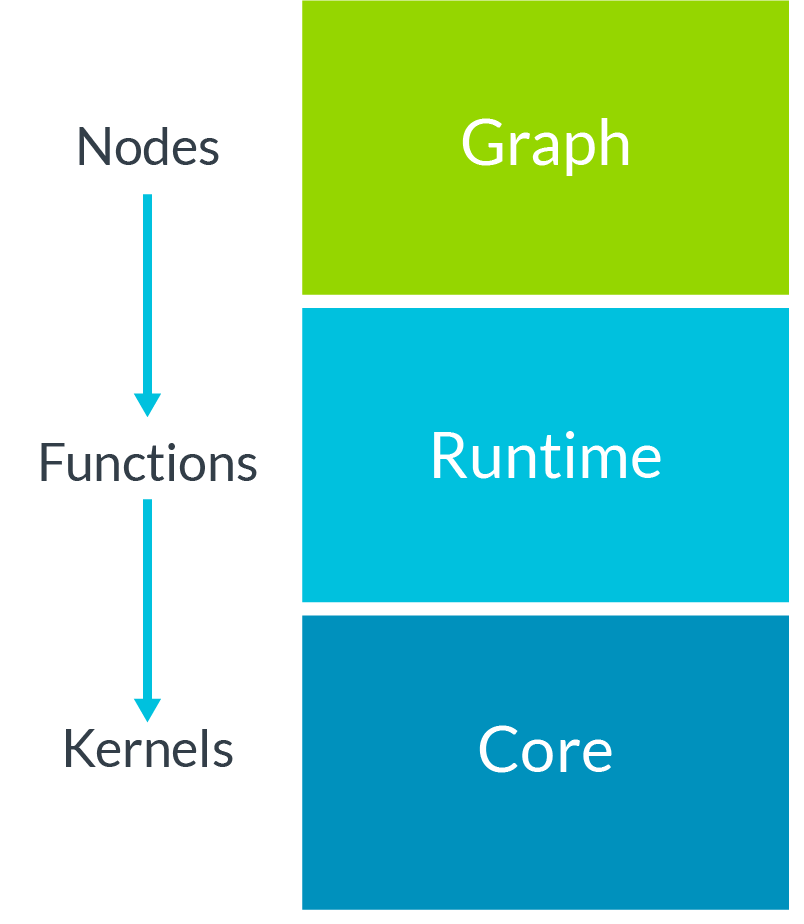## Introducing AlexNet

AlexNet is a convolutional neural network (CNN) that rose to prominence when it won the ImageNet Large Scale Visual Recognition Challenge (ILSVRC), an annual challenge that aims to evaluate algorithms for object detection and image classification. The model is trained on more than a million images and can classify images into 1000 object categories.

The ILSVRC evaluates the success of image classification solutions by using two important metrics, the top-5 and top-1 errors. When given a set of N images, often called test images, and mapped to a target class for each metric:

• top-1 error checks if the top predicted class is the same as the target class.
• top-5 error checks if the target class is one of the top five predictions.

For both metrics, the top error is calculated as, "the number of times the predicted class does not match the target class, divided by the total number of test images". In simpler terms, a lower score is better.

AlexNet achieved a top-5 error around 16%, which was an extremely good result back in 2012. To put it into context, until that year no other classifier had been able achieve results under 20%. AlexNet was also more than 10% more accurate than the runner up.

Since 2012, other CNNs, such as VGG and ResNet, have improved on AlexNet's performance, as illustrated in this graph.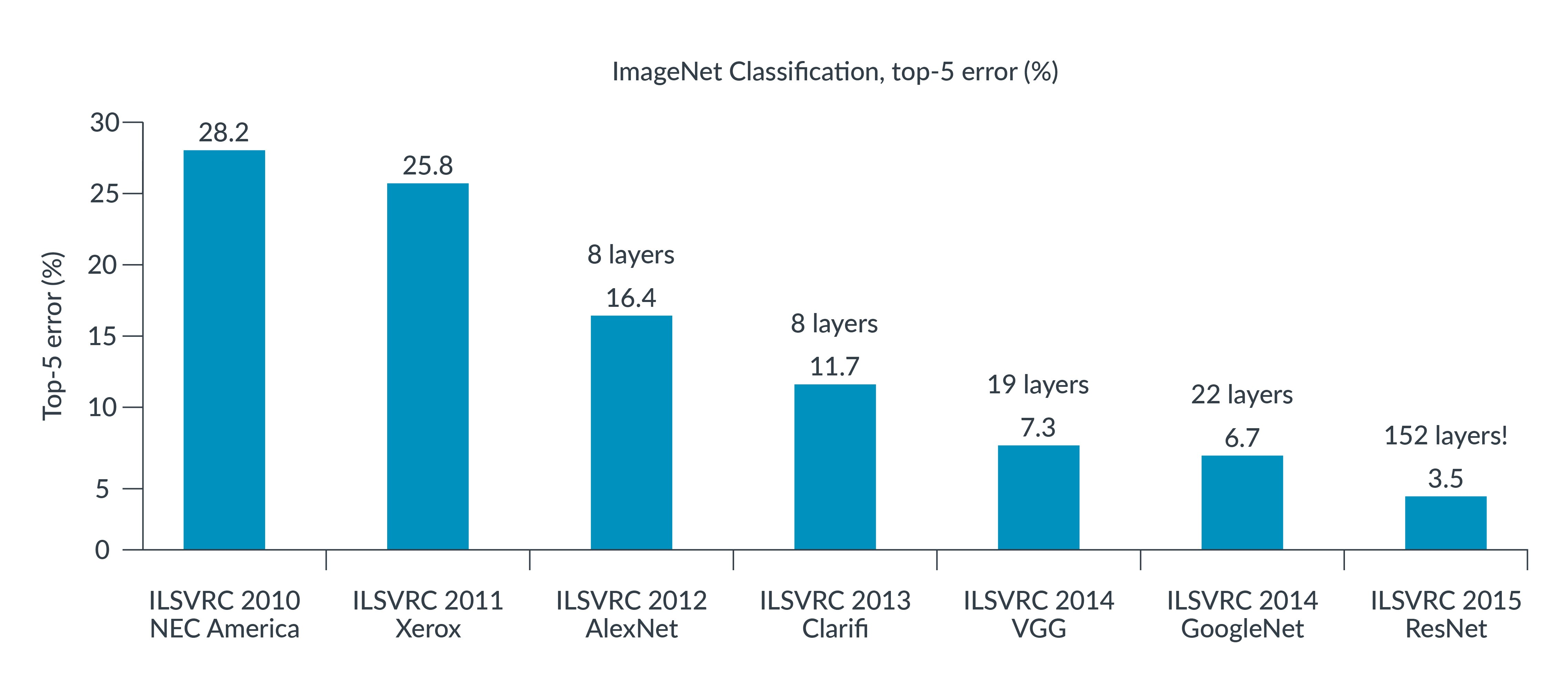##### What does AlexNet consist of?

AlexNet is made up of eight trainable layers, five convolution layers and three fully connected layers. All the trainable layers are followed by a ReLU activation function, except for the last fully connected layer, where the Softmax function is used.

Besides the trainable layers, the network also has:

• Three pooling layers.
• Two normalization layers.
• One dropout layer. This is only used for training to reduce the overfitting.

This table shows the layers and their details:

n. Layer  Info
1 Convolution 11x11x3x96 - (Stride(4,4) - Pad(0,0)
2 Activation ReLU
3 Normalization Cross Map, 5,0.0001,0.75
4 Pooling 3x3 - Stride(2,2)
5 Grouping Convolution  5x5x96x256 - Stride(1,1) - Pad(2,2)
6 Activation ReLU
7 Normalization  Cross Map, 5,0.0001,0.75
8 Pooling 3x3 - Stride(2,2)
9 Convolution 3x3x256x384 - Stride(1,1) - Pad(1,1)
10 Activation ReLU
11 Grouping Convolution 3x3x384x384 - Stride(1,2) - Pad(1,1)
12 Activation ReLU
13 Grouping Convolution 3x3x384x256 - Stride(1,1) - Pad(1,1)
14 Activation ReLU
15 Pooling 3x3 - Stride(2,2)
16 Fully connected 4096x9216 - Stride(1,1) - Pad(1,1)
17 Activation ReLU
18 Fully connected 4096x9216 - Stride(1,1) - Pad(1,1)
19 Activation ReLU
20 Fully connected 1000x4096 - Stride(1,1) - Pad(1,1)
21 Softmax
##### Grouping

In the table, there are some convolution layers that are actually grouping convolutions. This is an efficient engineering trick that allows the acceleration of the network over two GPUs without sacrificing accuracy.

If the group size is set to two, then the first half of the filters is connected to the first half of the input feature maps. The second half is connected to the second half of the input feature maps, as this image shows.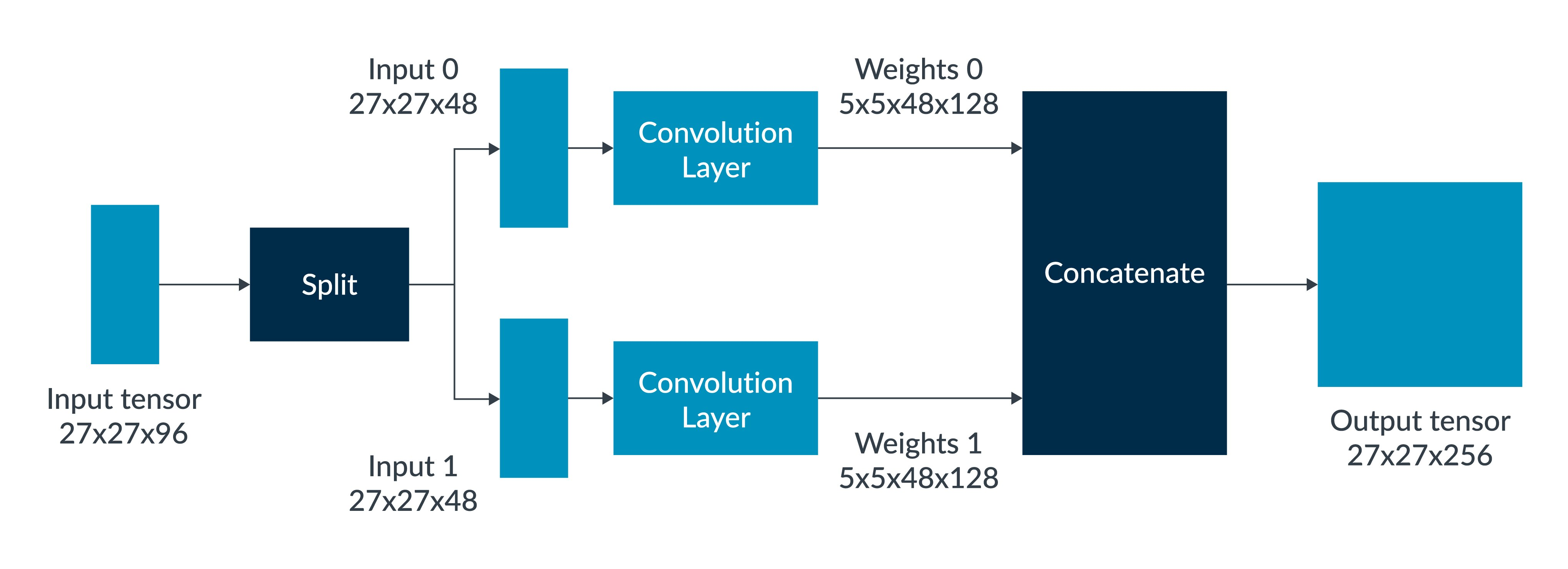The grouping convolution not only allows you to spread the workload over multiple GPUs, it also reduces the number of MACs needed for the layer by half.

## Evaluate the example code

A C++ implementation of AlexNet using the Graph API is proposed in examples/graph_alexnet.cpp. Here, we look at some key elements of the code and what they do.

To run the AlexNet example, you need to include these four command-line arguments:

```./graph_alexnet <target> <path_cnn_data> <input_image> <labels>
```

Where:

1. Target is the type of acceleration (`NEON=0` or `OpenCL=1`).
2. Path to cnn_data.
3. Path to your input image. Note that only .ppm files are supported).
4. Path to your ImageNet labels.

These subsections describe the key aspects of the example:

#### Mean subtraction pre-processing

A pre-processing stage is needed for preparing the input RGB image before feeding the network, so we are going to subtract the channel means from each individual color channel. This operation centers the red, green, and blue channels around the origin: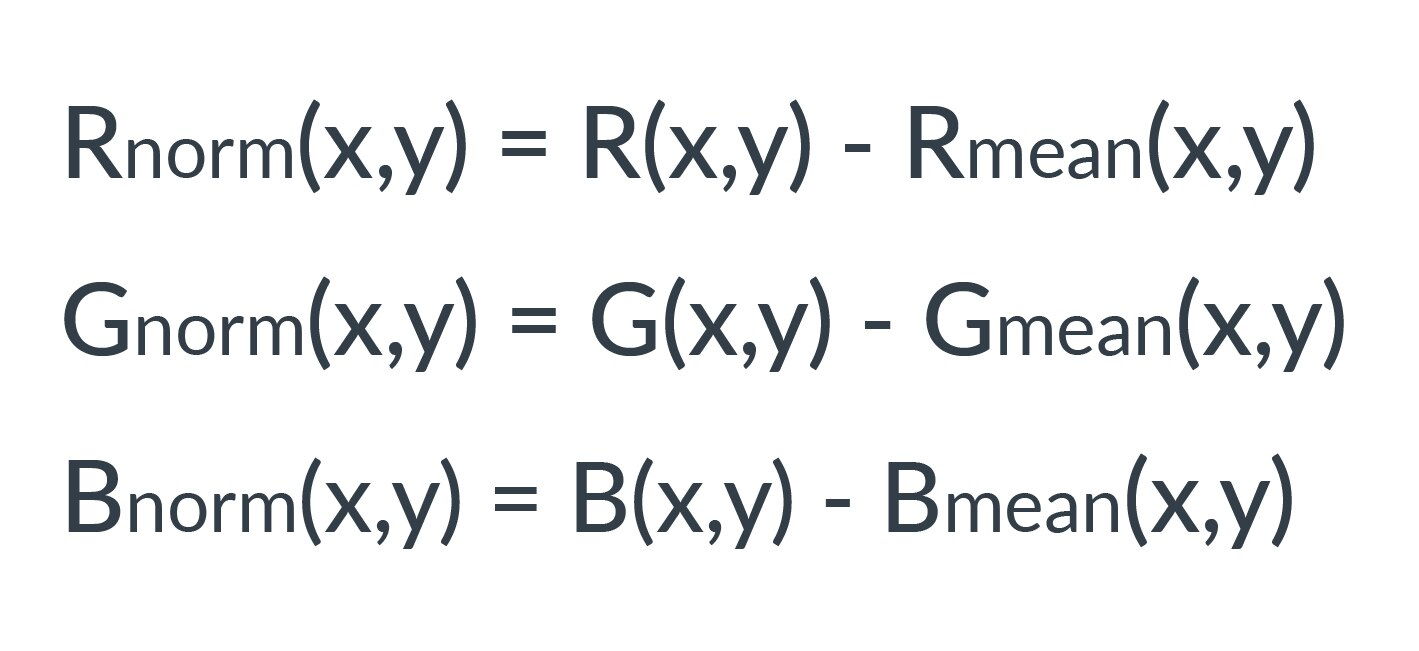Where:

• r_norm(x,y), g_norm(x,y), b_norm(x,y) are the RGB values at coordinates x,y after the mean subtraction.
• r(x,y), g(x,y), b(x,y) are the RGB values at coordinates x,y before the mean subtraction.
• r_mean, g_mean and b_mean are the mean values to use for the RGB channels.

For simplicity, the mean values for the examples are already hard-coded as:

`const std::array<float, 3=""> mean_rgb{ { 122.68f, 116.67f, 104.01f } }; </float,>`

If you are not familiar with mean subtraction pre-processing before, the Compute Image Mean section on the Caffe website provides a useful explanation.

#### Network description

The body of the network is described through the Graph API.

The graph consists of three main parts:

1. Mandatory: One input "Tensor object". This layer describes the geometry of the input data along with the data type to use. In this case, we will have a 3D input image with shape 227x227x3, using the FP32 data type.
2. The Convolution Neural Network layers, or ‘nodes’ in the graph's terminology. These are needed for the network.
3. Mandatory: One output "Tensor object". This is used to get the result back from the network.

As you can see in the example, the Tensor objects (input and output) and all of the trainable layers accept an input function called accessor.

Important: The accessor is the only way to access the internal Tensors.

• The accessor used by the input Tensor object can initialize the input Tensor of the network. This function can also be responsible for the mean subtraction pre-processing and reading the input image from a file or camera.
• The accessor used by the trainable layers, such as convolution, fully connected, and so on, can initialize the weights and the biases reading. For example, the values from a NumPy file.
• The accessor used by the output Tensor object can return the result of the classification, along with the score.

If you want to understand how the accessor works, see the utils/GraphUtils.h file. This has some ready-to-use accessors for your Tensor objects and trainable layers.

Here, you are going to turn on your Raspberry Pi and test AlexNet with some images.

First, turn on your Raspberry Pi and navigating to the home directory of your Raspberry Pi or host machine.

Then, on your Raspberry Pi enter the following commands:

```# Install unzip
sudo apt-get install unzip
# Download the zip file with the AlexNet model, input images and labels
wget https://developer.arm.com//-/media/Arm Developer Community/Images/Tutorial Guide Diagrams and Screenshots/Machine Learning/Running AlexNet on Pi with Compute Library/compute_library_alexnet.zip?revision=c1a232fa-f328-451f-9bd6-250b83511e01
# Create a new folder
mkdir assets_alexnet
# Unzip
unzip compute_library_alexnet.zip -d assets_alexnet ```

The contents of the ZIP file are now extracted into the assets_alexnet folder.

## Compile the Arm Compute Library

To compile natively on your Raspberry Pi, enter the following on the command-line:

```# Clone Compute Library
git clone https://github.com/Arm-software/ComputeLibrary.git
# Enter ComputeLibrary folder
cd ComputeLibrary
# Native Build the library and the examples
scons Werror=1 debug=0 asserts=0 neon=1 opencl=1 examples=1 build=native –j2 ```

Or, to cross-compile, enter the following on the command-line:

```# Clone Compute Library
git clone https://github.com/Arm-software/ComputeLibrary.git
# Enter ComputeLibrary folder
cd ComputeLibrary
# Build the library and the examples
scons Werror=1 debug=0 asserts=0 neon=1 opencl=1 examples=1 os=linux arch=armv7a -j4
# Copy the example and dynamic libraries on the Raspberry Pi
scp build/example/graph_alexnet build/libarm_compute.so build/libarm_compute_core.so build/libarm_compute_graph.so @:Desktop```

Where:

• `<username_raspberrypi>` is the username used on your Raspberry Pi.
• `<ip_addr_raspberrypi>` is the IP address of your Raspberry Pi.

## Run the classifier

The CNN has been trained to recognize 1000 object categories. A go-kart is one of these. This step demonstrates how the object is recognized when the CNN is passed an image of a go-kart. In contrast, if you defined a random image which is not part of the 1000 categories then the CNN will not be able to recognize it it.

If you compiled natively on your Raspberry Pi, enter the following on the command line to run the classifier against the go_kart.ppm image:

`./build/examples/graph_alexnet --data=\$PATH_ASSETS --image=\$PATH_ASSETS/go_kart.ppm --labels=\$PATH_ASSETS/labels.txt --target=neon –type=f32 –threads=4 `

Or, if you cross-compiled, on your host machine open an SSH session by entering:

`ssh <username_raspberrypi>@<ip_addr_raspberrypi>&gt;?</ip_addr_raspberrypi></username_raspberrypi>`

And in the SSH session, enter the Desktop folder and run the classifier against the go-kart .ppm image:

```cd Desktop
export LD_LIBRARY_PATH=build/
PATH_ASSETS=../assets_alexnet
./build/examples/graph_alexnet 0 \$PATH_ASSETS  \$PATH_ASSETS/go_kart.ppm \$PATH_ASSETS/labels.txt```

Whether or not you are building the library natively, the output should look like this if a successful classification has been performed: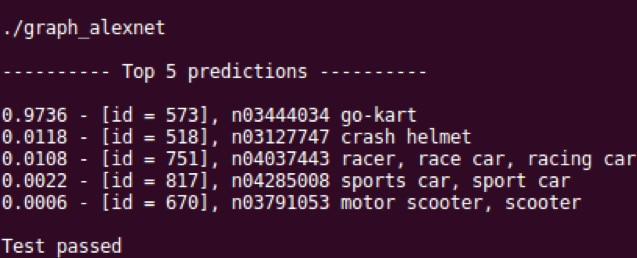This screenshot shows that the classifier has provided five predictions of the content of the image against the object categories that the CNN has been trained with. If the output does not look like the screenshot, then it is highly likely that the assets have not been correctly copied to the SD card.

## Develop your own network using the Arm Compute Library

If your test passed, then you have successfully used AlexNet to classify the characteristics of an image.

You can now go on to use what you have learned here to develop even more exciting and performant intelligent vision solutions on Arm-based platforms.

The Arm Compute Library contains other CNNs that are capable of recognizing objects with greater accuracy than AlexNet. These include MobileNet, VGG-16, and GoogleNet. Try experimenting with these as you begin to develop your own network using the Arm Compute Library.

## Related information

Arm Compute Library documentation

## Next steps

In this tutorial, we have learned how to run a model for image classification. Run the following command when to complete the guide.

• Run model for object detection (e.g. graph_ssd_mobilenet, graph_yolov3)